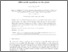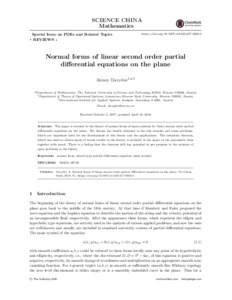# Normal forms of linear second order partial differential equations on the plane

Davydov, A. (2018). Normal forms of linear second order partial differential equations on the plane. Science China Mathematics 61 (11) 1947-1962. 10.1007/s11425-017-9303-0.Preview
Text
Davydov2018_Article_NormalFormsOfLinearSecondOrder.pdf - Published Version

## Abstract

The paper is devoted to the theory of normal forms of main symbols for linear second order partial differential equations on the plane. We discuss the results obtained in the last decades and some problems, which are important both for the development of this theory and the applications. The reduction theorem, which was used to obtain many of recent results in the theory, is included in the paper in the parametric form together with proof. There is a feeling that the theorem still has potential to get progress in the solution of open problems in the theory.

Item Type: Article normal form; mixed type partial differential equation; main symbol Advanced Systems Analysis (ASA) Luke Kirwan 28 Sep 2018 06:18 27 Aug 2021 17:30 http://pure.iiasa.ac.at/15489View Item